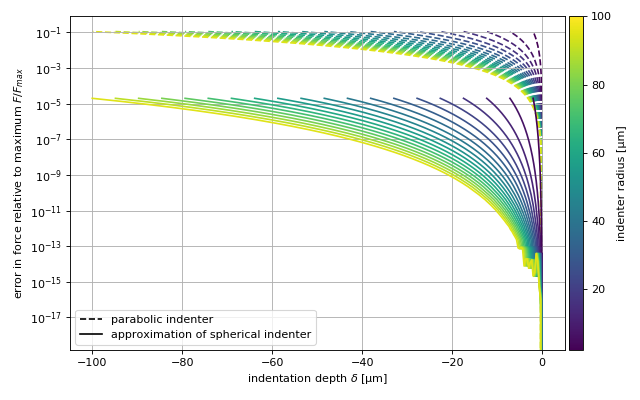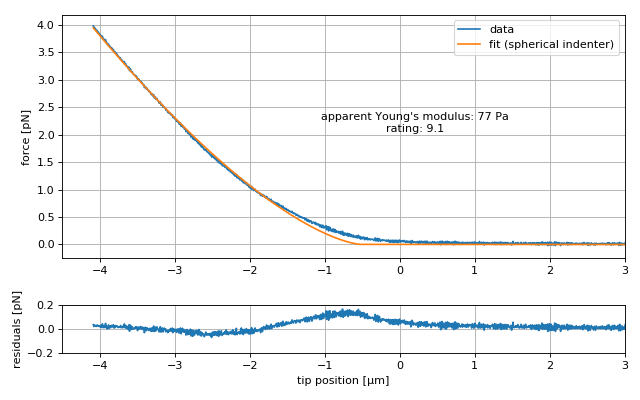# Scripting examples¶

## Approximating the Hertzian model with a spherical indenter¶

There is no closed form for the Hertzian model with a spherical indenter. The force $$F$$ does not directly depend on the indentation depth $$\delta$$, but has an indirect dependency via the radius of the circular contact area between indenter and sample $$a$$ [Sneddon1965]:

$\begin{split}F &= \frac{E}{1-\nu^2} \left( \frac{R^2+a^2}{2} \ln \! \left( \frac{R+a}{R-a}\right) -aR \right) \label{eq:1}\\ \delta &= \frac{a}{2} \ln \! \left(\frac{R+a}{R-a}\right) \label{eq:2}\end{split}$

Here, $$E$$ is the Young’s modulus, $$R$$ is the radius of the indenter, and $$\nu$$ is the Poisson’s ratio of the probed material.

Because of this indirect dependency, fitting this model to experimental data can be time-consuming. Therefore, it is beneficial to approximate this model with a polynomial function around small values of $$\delta/R$$ using the Hertz model for a parabolic indenter as a starting point [Dobler]:

$F = \frac{4}{3} \frac{E}{1-\nu^2} \sqrt{R} \delta^{3/2} \left(1 - \frac{1}{10} \frac{\delta}{R} - \frac{1}{840} \left(\frac{\delta}{R}\right)^2 + \frac{11}{15120} \left(\frac{\delta}{R}\right)^3 + \frac{1357}{6652800} \left(\frac{\delta}{R}\right)^4 \right)$

This example illustrates the error made with this approach. In nanite, the model for a spherical indenter has the identifier “sneddon_spher” and the approximate model has the identifier “sneddon_spher_approx”.

The plot shows the error for the parabolic indenter model “hertz_para” and for the approximation to the spherical indenter model. The maximum indentation depth is set to $$R$$. The error made by the approximation of the spherical indenter is more than four magnitudes lower than the maximum force during indentation.model_spherical_indenter.py

  1 2 3 4 5 6 7 8 9 10 11 12 13 14 15 16 17 18 19 20 21 22 23 24 25 26 27 28 29 30 31 32 33 34 35 36 37 38 39 40 41 42 43 44 45 46 47 48 49 50 51 52 53 54 55 56 57 58 59 60 61 62 63 import matplotlib.pylab as plt from mpl_toolkits.axes_grid1 import make_axes_locatable from matplotlib.lines import Line2D import matplotlib as mpl import numpy as np from nanite.model import models_available # models exact = models_available["sneddon_spher"] approx = models_available["sneddon_spher_approx"] para = models_available["hertz_para"] # parameters params = exact.get_parameter_defaults() params["E"].value = 1000 # radii radii = np.linspace(2e-6, 100e-6, 20) # plot results plt.figure(figsize=(8, 5)) # overview plot ax = plt.subplot() for ii, rad in enumerate(radii): params["R"].value = rad # indentation range x = np.linspace(0, -rad, 300) yex = exact.model(params, x) yap = approx.model(params, x) ypa = para.model(params, x) ax.plot(x*1e6, np.abs(yex - yap)/yex.max(), color=mpl.cm.get_cmap("viridis")(ii/radii.size), zorder=2) ax.plot(x*1e6, np.abs(yex - ypa)/yex.max(), ls="--", color=mpl.cm.get_cmap("viridis")(ii/radii.size), zorder=1) ax.set_xlabel(r"indentation depth $\delta$ [µm]") ax.set_ylabel("error in force relative to maximum $F/F_{max}$") ax.set_yscale("log") ax.grid() # legend custom_lines = [Line2D(, , color="k", ls="--"), Line2D(, , color="k", ls="-"), ] ax.legend(custom_lines, ['parabolic indenter', 'approximation of spherical indenter']) divider = make_axes_locatable(ax) cax = divider.append_axes("right", size="3%", pad=0.05) norm = mpl.colors.Normalize(vmin=radii*1e6, vmax=radii[-1]*1e6) mpl.colorbar.ColorbarBase(ax=cax, cmap=mpl.cm.viridis, norm=norm, orientation='vertical', label="indenter radius [µm]" ) plt.tight_layout() plt.show() 

## Fitting and rating¶

This example uses a force-distance curve of a zebrafish spinal cord section to illustrate basic data fitting and rating with nanite. The dataset is part of a study on spinal cord stiffness in zebrafish [Moellmert2019].fit_and_rate.py

  1 2 3 4 5 6 7 8 9 10 11 12 13 14 15 16 17 18 19 20 21 22 23 24 25 26 27 28 29 30 31 32 33 34 35 36 37 38 39 40 41 42 43 44 45 46 47 48 49 50 51 52 53 54 55 56 57 58 59 60 61 62 63 64 65 import matplotlib.gridspec as gridspec import matplotlib.pylab as plt import nanite # load the data group = nanite.load_group("data/zebrafish-head-section-gray-matter.jpk-force") idnt = group # this is an instance of nanite.Indentation # apply preprocessing idnt.apply_preprocessing(["compute_tip_position", "correct_force_offset", "correct_tip_offset"]) # set the fit model ("sneddon_spher_approx" is faster than "sneddon_spher" # and sufficiently accurate) idnt.fit_properties["model_key"] = "sneddon_spher_approx" # get the initial fit parameters params = idnt.get_initial_fit_parameters() # set the correct indenter radius params["R"].value = 18.64e-06 # perform the fit with the edited parameters idnt.fit_model(params_initial=params) # obtain the Young's modulus emod = idnt.fit_properties["params_fitted"]["E"].value # obtain a rating for the dataset # (using default regressor and training set) rate = idnt.rate_quality() # overview plot plt.figure(figsize=(8, 5)) gs = gridspec.GridSpec(2, 1, height_ratios=[5, 1]) ax1 = plt.subplot(gs) ax2 = plt.subplot(gs) # only plot the approach part (1 would be retract) where_approach = idnt["segment"] == 0 # plot force-distance data (nanite uses SI units) ax1.plot(idnt["tip position"][where_approach] * 1e6, idnt["force"][where_approach] * 1e9, label="data") ax1.plot(idnt["tip position"][where_approach] * 1e6, idnt["fit"][where_approach] * 1e9, label="fit (spherical indenter)") ax1.text(.2, 2.05, "apparent Young's modulus: {:.0f} Pa\n".format(emod) + "rating: {:.1f}".format(rate), ha="center") ax1.legend() # plot resiudals ax2.plot(idnt["tip position"][where_approach] * 1e6, (idnt["force"] - idnt["fit"])[where_approach] * 1e9) # update plot parameters ax1.set_xlim(-4.5, 3) ax1.set_ylabel("force [pN]") ax1.grid() ax2.set_xlim(-4.5, 3) ax2.set_ylim(-.2, .2) ax2.set_ylabel("residuals [pN]") ax2.set_xlabel("tip position [µm]") ax2.grid() plt.tight_layout() plt.show()